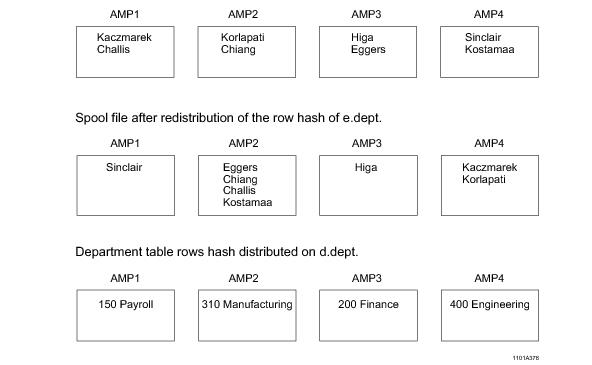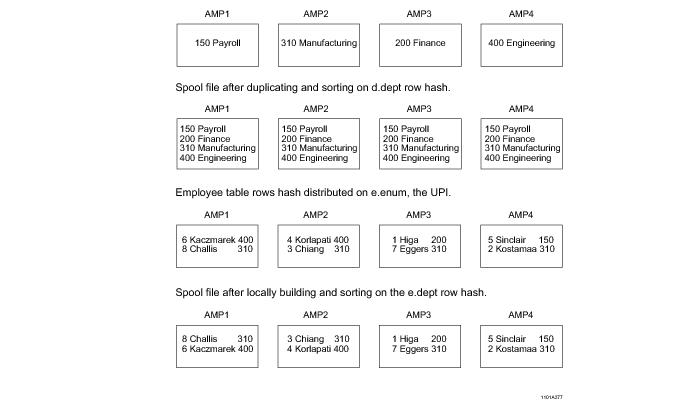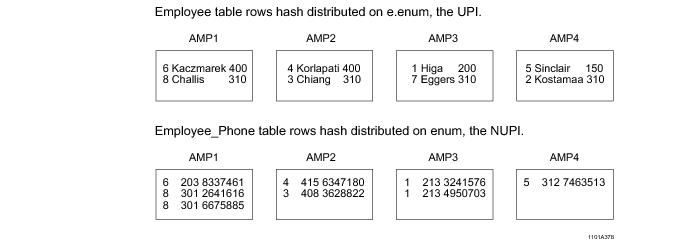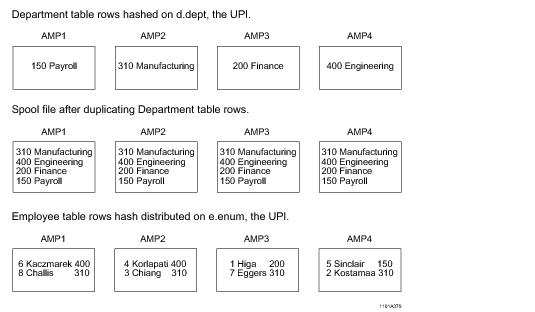# 15.10 - Join Geography - Teradata Database

## Teradata Database SQL Request and Transaction Processing

prodname
vrm_release
15.10
category
Programming Reference
User Guide
featnum
B035-1142-151K

Join geography is a term that describes the relocation, if any, of rows required to perform a join. Remember that for two rows to be joined, they must be on the same AMP, so the Optimizer must determine the least costly row relocation strategy, and the relative cardinalities of the two relations in any join is the principal cost that must be considered. This is one of the main factors that compels having fresh statistics available for the Optimizer.

Suppose a query joins tables a and b, and because these tables have different primary indexes, or because one table has a primary index and the other does not, the rows that must be joined are on different AMPs. Some sort of relocation of rows is required to make this join.

The last time statistics were collected on these tables, their respective cardinalities were 1,000 and 75,000 rows. These cardinalities clearly call for the Optimizer to relocate the few rows from table a to the AMPs containing the many rows of table b.

However, since statistics were last collected on these tables, unforeseen events have caused large shifts in the ratio of their cardinalities, and they are now 50,000 for table a and 3,500 for table b, as summarized by the following table:

 Table Cardinality When Statistics Last Collected Current Cardinality a 1,000 50,000 b 75,000 3,500

Based on the statistics available to it, the Optimizer will choose to relocate the rows of table a, which is clearly a far more costly relocation to make given the actual cardinalities of tables a and b today.

This is obviously an extreme example, but it illustrates why it is critical to keep fresh statistics on all of your frequently accessed tables.

The method the Optimizer chooses to use to distribute rows that are to be joined depends on several factors, but primarily on the availability of indexes and statistics. Keep the following facts about joins in mind:

• Join costs increase as a function of the number of rows that must be relocated, sorted, or both.
• Join plans for the same pairs of relations can change as a function of changes in the demographic properties of those relations.
• There are four possible join distribution strategies the Optimizer can choose among, and more than one strategy can be used for any join depending on circumstances. The four join distribution strategies are the following:

• Redistribution of one or both relations in the join.
• The EXPLAIN phrase to watch for is Redistribute.

• Duplication of one or both relations in the join.
• The EXPLAIN phrase to watch for is Duplicate.

• Locally building a spool copy of a relation.
• The EXPLAIN phrase to watch for is Locally Build.

• Sorting a relocated relation.
• The EXPLAIN phrase to watch for is Sort.

There is also a fifth option, which is not to redistribute any rows. This option represents the degenerate case of row redistribution where no redistribution is required to make the join, and it occurs only when the primary [AMP] indexes of the two relations are such that equal‑valued rows of both relations hash to the same AMPs.

The following set of examples demonstrates four different row redistribution strategies, three using merge join and a fourth using product join.

Note that with the exception of NoPI tables, the primary [AMP] index is the major consideration used by the Optimizer in determining how to join two relations and deciding which rows to relocate.

This example illustrates a merge join strategy, which includes redistributing the rows of one table and sorting them by the row hash value of the join column. A relocation strategy is pursued because the join condition in the query is on only one of the primary indexes of the joined tables (see “Scenario 2: Join Column Set is the Primary Index of Only One of the Tables in the Join” on page 373).

The query that generates a merge join is the following.

`    SELECT *`
`    FROM employee AS e INNER JOIN department AS d`
`    ON e.dept = d.dept;`

The two tables to be joined are defined as follows:

The following graphic shows how individual rows would be relocated to make this join for a 4‑AMP system:The example is run on a 4‑AMP system. The query uses an equijoin condition on the dept columns of both tables. The system copies the employee table rows into spool and redistributes them on the row hash of e.dept. The merge join occurs after the rows to be joined have been relocated so they are on the same AMPs.

The relocation strategy occurs when one of the tables is already distributed on the join column row hash. The merge join is caused by the join column being the primary index of one (dept), but not both, of the tables.

This example illustrates a different merge join strategy that consists of duplicating and sorting the smaller table in the join on all AMPs and locally building a copy of the larger table and sorting it on the employee table row hash (see “Scenario 2: Join Column Set is the Primary Index of Only One of the Tables in the Join” on page 373).

This query and tables used for this example are the same as those used for “Redistributing the Rows of One Table for a Merge Join” on page 366, but the Optimizer pursues a different join geography strategy because the statistics for the tables are different. If the Optimizer determines from the available statistics that it would be less expensive to duplicate and sort the smaller table than to hash redistribute the larger table, it chooses the strategy followed by this scenario.

The query that generates a merge join is the following.

`    SELECT *`
`    FROM employee AS e INNER JOIN department AS d`
`    ON e.dept = d.dept;`

The two tables to be joined are defined as follows:

The following graphic shows how individual rows would be relocated to make this join for a 4‑AMP system:The system first duplicates the department table and then sorts it on the dept column for all AMPs. Next, the employee table is built locally and sorted on the row hash value of dept.

The final step in the process is to perform the actual merge join operation.

This example also illustrates a merge join strategy. This particular strategy does not require any duplication or sorting of rows because the joined rows of both tables hash to the same AMPs. This is a good example of using a NUPI for one table on the same domain as the UPI of the other table. This ensures that the rows having the same primary index values all hash to the same AMP (see “Scenario 1: Join Column Set is the Primary Index of Both Relations in the Join” on page 372). When the join condition is on those primary indexes, the rows to be joined are already on the same AMP, so no relocation or sorting need be done. All the system has to do is compare the rows that are already on the proper AMPs.

This example is run on a 4-AMP System.

The query that generates this merge join strategy is the following:

`    SELECT *`
`    FROM employee AS e INNER JOIN employee_phone AS p`
`    ON e.num = p.num;`

The two tables to be joined are defined as follows.

The following graphic shows how individual rows would be relocated to make this join for a 4‑AMP system:This example illustrates a product join strategy, which includes duplicating the smaller table rows on every AMP of a 4‑AMP system. The tables used are the same as those used in “Scenario 2: Join Column Set is the Primary Index of Only One of the Tables in the Join” on page 373, and the query is the same as well with the exception that the join condition in that scenario is an equijoin, while the join condition in this query is a θ join, where θ is >.

The query that generates a product join is the following.

`    SELECT *`
`    FROM employee AS e INNER JOIN department AS d`
`    ON e.dept > d.dept;`

The two tables to be joined are defined as follows:

The product join is caused by the non‑equijoin condition `e.dept > d.dept` (see “Product Join” on page 398). Based on the available statistics, the Optimizer determines that the department table is the smaller table in the join, so it devises a join plan that distributes copies of all those rows to each AMP. employee table rows, which are hash distributed on their UPI enum values, are not relocated. The product join operation returns only the rows that satisfy the specified join condition for the query after comparing every row in the employee table with every row in the duplicated department table.

The following graphic shows how individual rows are relocated to make this join for the 4‑AMP system.The primary [AMP] index is the major consideration used by the Optimizer in determining how to join two tables and deciding which rows to relocate, though for tables that have no primary index, other criteria must be considered.

Three general scenarios can occur when two primary‑indexed relations are joined using the merge join method (see “Merge Join” on page 405), as illustrated by the following table.

The scenarios are ranked in order of best to worst, with 1 being best, where best means least costly:

 Rank Scenario WHEN the join column set is … FOR these relations in the join operation … 1 1 the primary [AMP] index both. 2 2 the primary [AMP] index one. 3 3 not the primary [AMP] index neither.

The three scenarios are described for a primary index in the topics that follow.

Note: The examples in these sections assume that all join columns come from the same domain.

This is the best case scenario because the rows to be joined are already located on the same AMP. Equal primary index values always hash to the same AMP, so there is no need to relocate rows to other AMPs. The rows are also already sorted in row hash order because of the way the file system stores them. With no need to sort or relocate rows, the join can be performed immediately with very little cost.

For example, consider the following query:

`    SELECT …`
`    FROM table_1 AS t1 INNER JOIN table_2 AS t2 `
`    ON t1.col_1=t2.col_1;`

The join in this query is defined on the primary index columns for both tables, as you can see from the example tables presented below. Because the primary index values for the rows are equal, they hash to the same AMP. The result is that no row relocation is required to make the join.

In this case, only one table has its rows on the target AMPs. The rows of the second table must be redistributed to their target AMPs by the hash code of the join column value. If the table is small (a small table is defined as a table whose cardinality is less than 5 times the number of AMPs in the system. For a 20‑AMP system, table cardinality would have to be less than 100 rows for the table to be considered small, for a 100‑AMP system, less than 500 rows, and so on), the Optimizer might decide to simply duplicate the entire table on all AMPs instead of hash redistributing individual rows. In either case, the system copies some or all of the rows from one table to their target AMPs. If the PI table is the smaller table, the Optimizer might choose to duplicate it on all AMPs rather than redistributing the non‑primary indexed table.

For example, consider the following query.

`    SELECT …`
`    FROM table_3 AS t3 INNER JOIN table_4 AS t4 `
`    ON t3.col_1=t4.col_2;`

The join in this query is defined on the primary index column for table_3 and on a non‑primary index column for table_4, as you can see from the example relations presented below. Because of this, the rows for one of the tables must be relocated to make the join.

In the example, the rows from table_4 are chosen for relocation to their target AMPs by hash code and placed into a spool, probably because the joined row cardinality of table_4 is much smaller than that of table_3. There is no rule that forces the rows of the non‑primary indexed table to be relocated. The decision depends entirely on the available statistics for both relations in the join.

If the joined row cardinality of table_4 is significantly smaller than that of table_3, the Optimizer might even decide to duplicate the entire smaller table rather than hash redistributing a subset of its rows.

This is the worst case scenario because if neither column is a primary index, then the rows of both must be redistributed to their target AMPs. This can be done either by hash redistribution of the join column value or by duplicating the smaller table on each AMP. In either case, this approach involves the maximum amount of data movement. Your choice of a primary index should be heavily influenced by the amount of join activity you anticipate for the table.

For example, consider the following query.

`    SELECT …`
`    FROM table_5 AS t5 INNER JOIN table_6 AS t6 `
`    ON t5.col_2=t6.col_3;`

The join in this query is defined on the unindexed col_2 of table_5 and on the unindexed col_3 of table_4, as you can see from the example relations presented below. Because of this, the rows for both relations must be relocated to their target AMPs to make the join.

In the example, the rows from both table_5 and table_6 are placed in spools so they can be relocated to their target AMPs by hash code. If the joined row cardinality of one of the relations is significantly smaller than that of the other, the Optimizer might even decide to duplicate the entire smaller table rather than hash redistributing a subset of its rows.